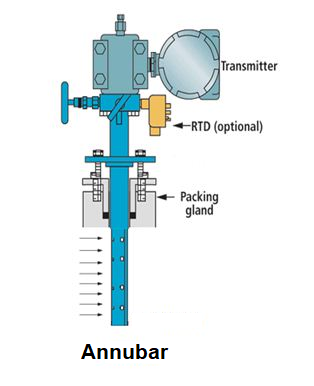Flow Measurement

# Annubar flowmeter operationAnnubars are sometimes called average pilots and contain multiple pressure taps to “average” the flow; this is to try to compensate for a non-ideal flow profile.

The averaged pitot tube is inserted through the pipe as shown below. One side of the bar has pressure taps facing the flowing fluid and engaging in an “average” chamber that measures the total (ie, static + dynamic) pressure of the fluid.There may be a single port or multiple outlet ports on the opposite side of the bar to measure the low static pressure in the downstream region.

The difference between the total pressure and the static pressure is effectively a measure of the height of the fluid velocity, which together with the area of the pipeline allows determining the volumetric flow.## Principle of Annubar operation:

The Annubar primary flow element is a device that is used to measure the flow of a liquid, gas or vapor that flows through a pipeline. It allows flow measurement by creating a differential pressure (DP) that is proportional to the square of the fluid velocity in the pipeline, according to Bernoulli’s theorem. This DP is measured and converted to a flow rate using a secondary device, such as a DP pressure transmitter.

The flow is related to DP through the following relationship.where:

Q = Flow Rate

K = Annubar Flow Coefficient

DP = Differential Pressure

The Annubar generates a DP by creating a blockage in the pipeline and acting as an obstruction to the fluid. The fluid velocity decreases and stops as it reaches the front surface of the Annubar sensor, creating the impact / high pressure.

The Annubar detects the impact pressure using a DP transmitter.As the fluid continues around the Annubar sensor, it creates a lower velocity profile on the back of the sensor, creating the low / suction pressure downstream of the Annubar. The individual ports, located on the back of the Annubar sensor, measure this low pressure. Working on the same principle as high pressure, an average low pressure is maintained at the low pressure that is connected directly to the transmitter for measurement.

The resulting differential pressure is the difference between the impact pressure reading (high) and the suction pressure reading (low) as seen below.

DP = PH – PL

where:

PH = High Pressure

PL = Low Pressure

The measured DP is used to calculate the flow rate.

• It can be inserted through a small opening.
• It can be used to sample the velocity at several points.
• Minimum obstruction that means less pressure drop